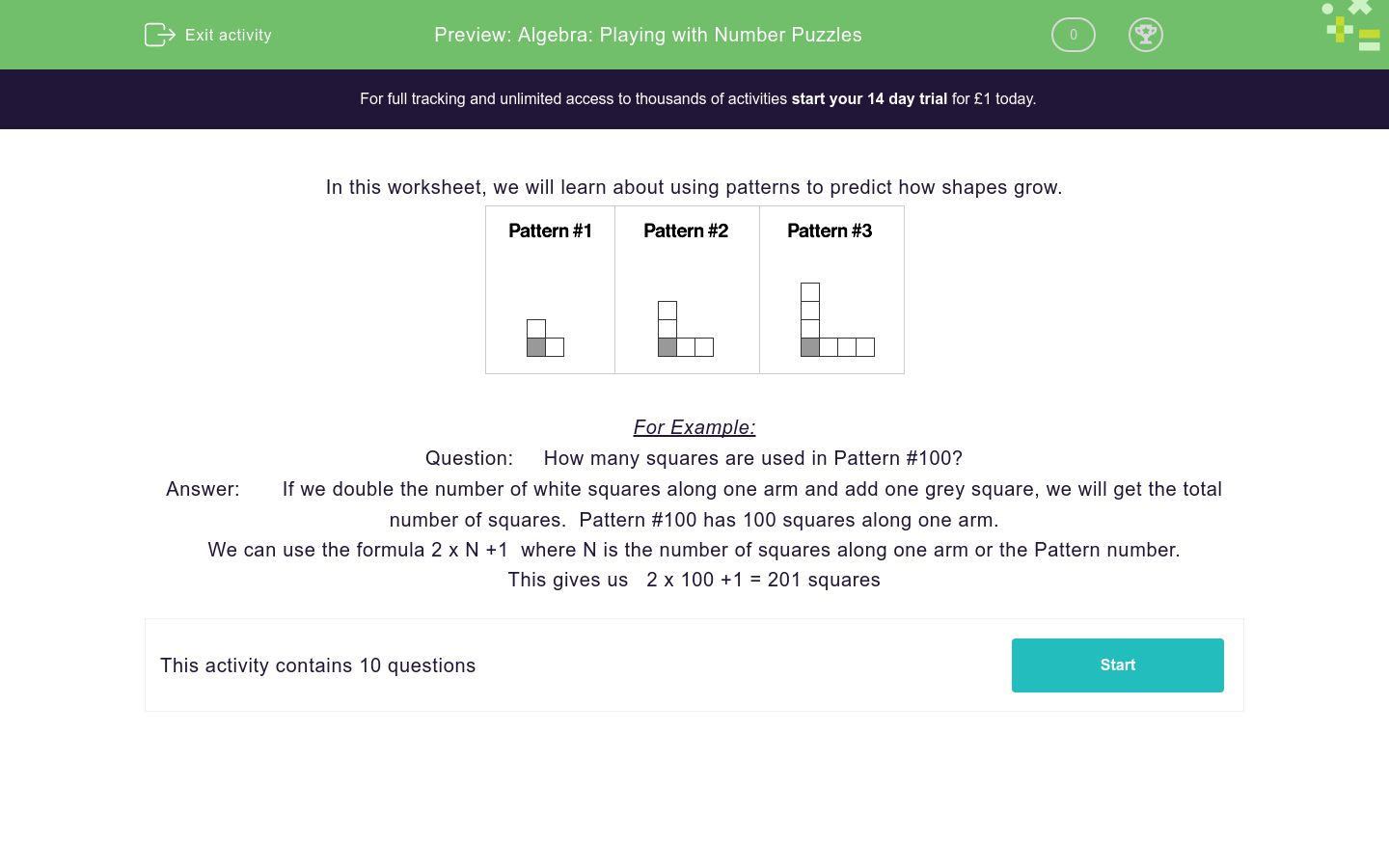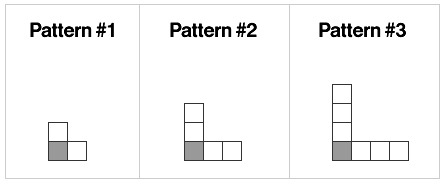# Algebra: Playing with Number Puzzles

In this worksheet, students solve number problems by working algebraically.Key stage:  KS 2

Curriculum topic:   Algebra

Curriculum subtopic:   Express Problems Algebraically

Difficulty level:### QUESTION 1 of 10

In this worksheet, we will learn about using patterns to predict how shapes grow.For Example:

Question:     How many squares are used in Pattern #100?

Answer:       If we double the number of white squares along one arm and add one grey square, we will get the total number of squares.  Pattern #100 has 100 squares along one arm.

We can use the formula 2 x N +1  where N is the number of squares along one arm or the Pattern number.

This gives us   2 x 100 +1 = 201 squaresHow many squares are in Pattern #21 ?How many squares are in Pattern #29 ?How many squares are in Pattern #72 ?How many squares are in Pattern #61 ?How many squares are in Pattern #53 ?How many squares are in Pattern #75 ?How many squares are in Pattern #86 ?How many squares are in Pattern #96 ?How many squares are in Pattern #48 ?How many squares are in Pattern #39 ?

• Question 1How many squares are in Pattern #21 ?

43
EDDIE SAYS
Use the formula 2 x N + 1 where N is the number of squares along one arm or the Pattern number. This gives us 2 x 21 + 1 = 43 squares.
• Question 2How many squares are in Pattern #29 ?

59
EDDIE SAYS
Use the formula 2 x N + 1 where N is the number of squares along one arm or the Pattern number. This gives us 2 x 29 + 1 = 59 squares.
• Question 3How many squares are in Pattern #72 ?

145
EDDIE SAYS
Use the formula 2 x N + 1 where N is the number of squares along one arm or the Pattern number. This gives us 2 x 72 + 1 = 145 squares.
• Question 4How many squares are in Pattern #61 ?

123
EDDIE SAYS
Use the formula 2 x N + 1 where N is the number of squares along one arm or the Pattern number. This gives us 2 x 61 + 1 = 123 squares.
• Question 5How many squares are in Pattern #53 ?

107
EDDIE SAYS
Use the formula 2 x N + 1 where N is the number of squares along one arm or the Pattern number. This gives us 2 x 53 + 1 = 107 squares.
• Question 6How many squares are in Pattern #75 ?

151
EDDIE SAYS
Use the formula 2 x N + 1 where N is the number of squares along one arm or the Pattern number. This gives us 2 x 75 + 1 = 151 squares.
• Question 7How many squares are in Pattern #86 ?

173
EDDIE SAYS
Use the formula 2 x N + 1 where N is the number of squares along one arm or the Pattern number. This gives us 2 x 86 + 1 = 173 squares.
• Question 8How many squares are in Pattern #96 ?

193
EDDIE SAYS
Use the formula 2 x N + 1 where N is the number of squares along one arm or the Pattern number. This gives us 2 x 96 + 1 = 193 squares.
• Question 9How many squares are in Pattern #48 ?

97
EDDIE SAYS
Use the formula 2 x N + 1 where N is the number of squares along one arm or the Pattern number. This gives us 2 x 48 + 1 = 97 squares.
• Question 10How many squares are in Pattern #39 ?

79
EDDIE SAYS
Use the formula 2 x N + 1 where N is the number of squares along one arm or the Pattern number. This gives us 2 x 39 + 1 = 79 squares.
---- OR ----

Sign up for a £1 trial so you can track and measure your child's progress on this activity.

### What is EdPlace?

We're your National Curriculum aligned online education content provider helping each child succeed in English, maths and science from year 1 to GCSE. With an EdPlace account you’ll be able to track and measure progress, helping each child achieve their best. We build confidence and attainment by personalising each child’s learning at a level that suits them.

Get started